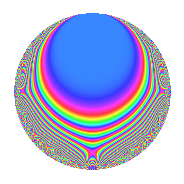# Properties

 Label 29.2.eLevel 29 Weight 2 Character orbit e Rep. character $$\chi_{29}(4,\cdot)$$ Character field $$\Q(\zeta_{14})$$ Dimension 12 Newforms 1 Sturm bound 5 Trace bound 0

# Related objects

## Defining parameters

 Level: $$N$$ = $$29$$ Weight: $$k$$ = $$2$$ Character orbit: $$[\chi]$$ = 29.e (of order $$14$$ and degree $$6$$) Character conductor: $$\operatorname{cond}(\chi)$$ = $$29$$ Character field: $$\Q(\zeta_{14})$$ Newforms: $$1$$ Sturm bound: $$5$$ Trace bound: $$0$$

## Dimensions

The following table gives the dimensions of various subspaces of $$M_{2}(29, [\chi])$$.

Total New Old
Modular forms 24 24 0
Cusp forms 12 12 0
Eisenstein series 12 12 0

## Trace form

 $$12q$$ $$\mathstrut -\mathstrut 7q^{2}$$ $$\mathstrut -\mathstrut 7q^{3}$$ $$\mathstrut -\mathstrut q^{4}$$ $$\mathstrut -\mathstrut q^{5}$$ $$\mathstrut -\mathstrut 3q^{6}$$ $$\mathstrut -\mathstrut 11q^{7}$$ $$\mathstrut +\mathstrut 14q^{8}$$ $$\mathstrut -\mathstrut 3q^{9}$$ $$\mathstrut +\mathstrut O(q^{10})$$ $$12q$$ $$\mathstrut -\mathstrut 7q^{2}$$ $$\mathstrut -\mathstrut 7q^{3}$$ $$\mathstrut -\mathstrut q^{4}$$ $$\mathstrut -\mathstrut q^{5}$$ $$\mathstrut -\mathstrut 3q^{6}$$ $$\mathstrut -\mathstrut 11q^{7}$$ $$\mathstrut +\mathstrut 14q^{8}$$ $$\mathstrut -\mathstrut 3q^{9}$$ $$\mathstrut -\mathstrut 7q^{10}$$ $$\mathstrut +\mathstrut 7q^{11}$$ $$\mathstrut +\mathstrut 9q^{13}$$ $$\mathstrut -\mathstrut 7q^{14}$$ $$\mathstrut +\mathstrut 7q^{15}$$ $$\mathstrut +\mathstrut 9q^{16}$$ $$\mathstrut +\mathstrut 42q^{18}$$ $$\mathstrut -\mathstrut 7q^{19}$$ $$\mathstrut -\mathstrut 11q^{20}$$ $$\mathstrut -\mathstrut 7q^{21}$$ $$\mathstrut -\mathstrut 4q^{22}$$ $$\mathstrut -\mathstrut 5q^{23}$$ $$\mathstrut -\mathstrut 25q^{24}$$ $$\mathstrut +\mathstrut 13q^{25}$$ $$\mathstrut -\mathstrut 21q^{26}$$ $$\mathstrut -\mathstrut 7q^{27}$$ $$\mathstrut +\mathstrut 12q^{28}$$ $$\mathstrut -\mathstrut 15q^{29}$$ $$\mathstrut +\mathstrut 2q^{30}$$ $$\mathstrut -\mathstrut 21q^{31}$$ $$\mathstrut -\mathstrut 17q^{33}$$ $$\mathstrut -\mathstrut 13q^{34}$$ $$\mathstrut +\mathstrut 19q^{35}$$ $$\mathstrut -\mathstrut 40q^{36}$$ $$\mathstrut +\mathstrut 7q^{37}$$ $$\mathstrut +\mathstrut 28q^{38}$$ $$\mathstrut +\mathstrut 21q^{39}$$ $$\mathstrut +\mathstrut 35q^{40}$$ $$\mathstrut +\mathstrut 50q^{42}$$ $$\mathstrut +\mathstrut 7q^{43}$$ $$\mathstrut +\mathstrut 42q^{44}$$ $$\mathstrut +\mathstrut 16q^{45}$$ $$\mathstrut -\mathstrut 7q^{47}$$ $$\mathstrut -\mathstrut 14q^{48}$$ $$\mathstrut +\mathstrut 13q^{49}$$ $$\mathstrut -\mathstrut 28q^{50}$$ $$\mathstrut +\mathstrut 20q^{51}$$ $$\mathstrut -\mathstrut 6q^{52}$$ $$\mathstrut -\mathstrut 10q^{53}$$ $$\mathstrut -\mathstrut 38q^{54}$$ $$\mathstrut -\mathstrut 35q^{55}$$ $$\mathstrut -\mathstrut 21q^{56}$$ $$\mathstrut -\mathstrut 14q^{57}$$ $$\mathstrut -\mathstrut 57q^{58}$$ $$\mathstrut +\mathstrut 44q^{59}$$ $$\mathstrut -\mathstrut 28q^{60}$$ $$\mathstrut -\mathstrut 7q^{61}$$ $$\mathstrut +\mathstrut 37q^{62}$$ $$\mathstrut -\mathstrut 13q^{63}$$ $$\mathstrut -\mathstrut 26q^{64}$$ $$\mathstrut -\mathstrut 6q^{65}$$ $$\mathstrut +\mathstrut 21q^{66}$$ $$\mathstrut -\mathstrut 37q^{67}$$ $$\mathstrut +\mathstrut 14q^{68}$$ $$\mathstrut +\mathstrut 21q^{69}$$ $$\mathstrut -\mathstrut 21q^{71}$$ $$\mathstrut +\mathstrut 35q^{72}$$ $$\mathstrut +\mathstrut 14q^{73}$$ $$\mathstrut +\mathstrut 7q^{76}$$ $$\mathstrut -\mathstrut 7q^{77}$$ $$\mathstrut +\mathstrut 17q^{78}$$ $$\mathstrut +\mathstrut 49q^{79}$$ $$\mathstrut -\mathstrut 6q^{80}$$ $$\mathstrut +\mathstrut q^{81}$$ $$\mathstrut +\mathstrut 22q^{82}$$ $$\mathstrut +\mathstrut 5q^{83}$$ $$\mathstrut +\mathstrut 21q^{84}$$ $$\mathstrut +\mathstrut 14q^{85}$$ $$\mathstrut -\mathstrut 44q^{86}$$ $$\mathstrut +\mathstrut 15q^{87}$$ $$\mathstrut -\mathstrut 66q^{88}$$ $$\mathstrut +\mathstrut 7q^{89}$$ $$\mathstrut +\mathstrut 28q^{90}$$ $$\mathstrut -\mathstrut 3q^{91}$$ $$\mathstrut -\mathstrut 6q^{92}$$ $$\mathstrut +\mathstrut 19q^{93}$$ $$\mathstrut +\mathstrut 66q^{94}$$ $$\mathstrut -\mathstrut 7q^{95}$$ $$\mathstrut +\mathstrut 30q^{96}$$ $$\mathstrut +\mathstrut 14q^{97}$$ $$\mathstrut -\mathstrut 42q^{98}$$ $$\mathstrut +\mathstrut O(q^{100})$$

## Decomposition of $$S_{2}^{\mathrm{new}}(29, [\chi])$$ into irreducible Hecke orbits

Label Dim. $$A$$ Field CM Traces $q$-expansion
$$a_2$$ $$a_3$$ $$a_5$$ $$a_7$$
29.2.e.a $$12$$ $$0.232$$ 12.0.$$\cdots$$.1 None $$-7$$ $$-7$$ $$-1$$ $$-11$$ $$q+(-1+\beta _{3}+\beta _{7}+\beta _{9}+\beta _{10})q^{2}+\cdots$$